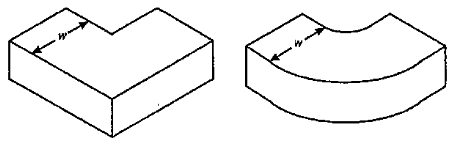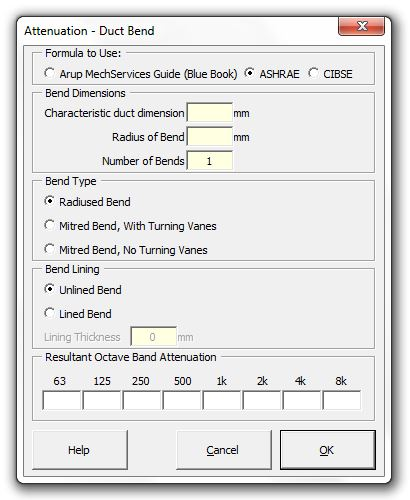### Strutt Help

Attenuation - Duct Bend    1/1

Strutt|Mechanical Services|Duct Elements|Bend inserts attenuation due to bends in ductwork into the active row of the worksheet.

Insertion loss data can be sourced from the 2019 ASHRAE Handbook - HVAC Applications (Tables 22, 23 and 24) or the Arup Sound and Vibration in Mechanical Services Design (Blue Book - Tables 14, 15 and 16).

The input required is the characteristic dimension of the duct, width (w) in the same plane as the change in direction of the duct, number of bends and the type of bend (plus the lining thickness, for some calculations).Arup Mechanical Services Guide is based on the Sound Research Laboratories book "Noise Control in Building Services" and references octave band attenuation according to the range that the duct width is within.

ASHRAE calculates the attenuation for bends as follows:

• For unlined or lined mitred bends without turning vanes, Strutt calculates based on the product of frequency and bend dimension f*d using the values in Table 22 ASHRAE 2019
• For unlined or lined mitred bends with turning vanes, Strutt calculates based on the product of frequency and bend dimension f*d using the values in Table 24 ASHRAE 2019
• For radiused bends, Strutt calculates based on the product of frequency and bend dimension f*d using the values in Table 23 ASHRAE 2019
• The ASHRAE handbook does not provide an alternative method for calculating the insertion loss of lined radiused bends. ASHRAE 1991 provided the following formulae (equations 5.19 and 5.20) for calculating the insertion loss for lined circular radiused bends. These formulae are not implemented in the current version of Strutt, as they are not well supported and have been removed from the ASHRAE guide since 1995:

For duct sizes between 6 and 18 inches (150 mm and 450 mm):
IL*(d/r)^2=0.485+2.094log(f*d)+3.172[log(fd)]^2-1.578[log(fd)]^4+0.085[log(fd)]^7

For duct sizes between 18 and 60 inches (450 mm and 1500 mm):
IL*(d/r)^2=-1.493+0.538t+1.406log(fd)+2.779[log(fd)]^2-0.662[log(fd)]^4+0.016[log(fd)]^7

where:
f is the frequency (Hz)
d is the duct diameter (in)
r is the radius of the bend (in)
t is the thickness of the duct lining (in)

CIBSE calculates the attenuation for bends as follows:

• For unlined or lined mitred bends without turning vanes, Strutt calculates based on the product of frequency and bend dimension f*d using the values in Table 5.6 CIBSE
• For unlined or lined mitred bends with turning vanes, Strutt calculates based on the product of frequency and bend dimension f*d using the values in Table 5.7 CIBSE
• For unlined radiused bends, Strutt calculates based on the product of frequency and bend dimension f*d using the values in Table 5.8 CIBSE
• For lined radiused bends, Strutt uses the following attenuation values (independent of the bend dimension)

 Lining 63 Hz 125 Hz 250 Hz 500 Hz 1 kHz 2 kHz 4 kHz 8 kHz 25 mm 0 2 5 7 10 10 10 10 50 mm 0 5 8 10 13 13 13 13
Note that the values for lined radiused ducts are approximate and should be used as an order of magnitude guide.

References:

• Arup, Sound and Vibration in Mechanical Services Design, pp41 - 43
• 2019 ASHRAE Handbook - HVAC Applications (Tables 22, 23 and 24)
• CIBSE Guide B 2005 Section 5.6.4